• Lanteria HR
Competency Score Calculations
• 25 May 2023
• Dark
Light

# Competency Score Calculations

• Dark
Light

Article Summary

After the competencies are assessed, the Total Score (displayed for all the competencies) and Requirement Met score (displayed for each competency group) are calculated.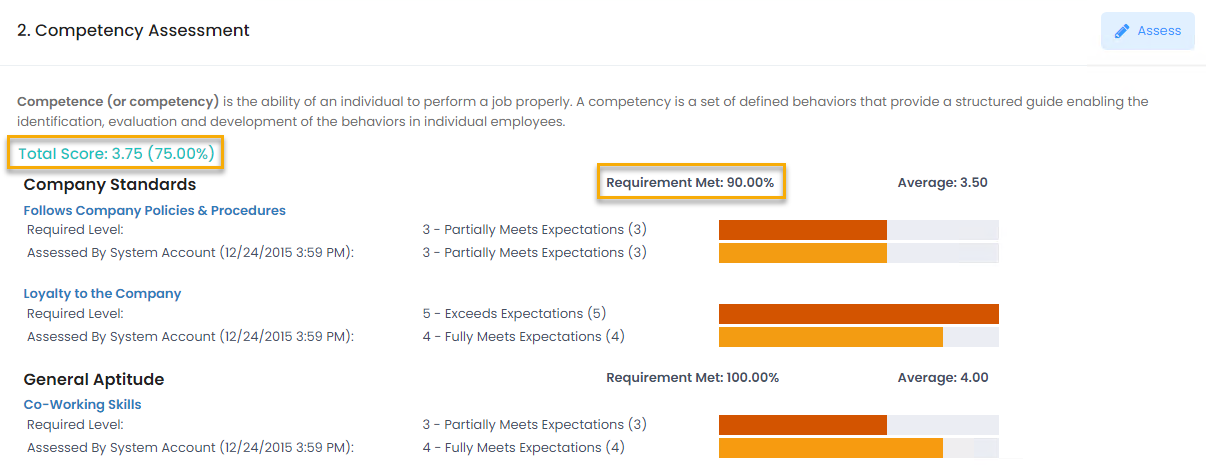## Total Score

The competencies are assessed by the Competency Levels defined under Settings > Settings and Configuration > Performance > Competency.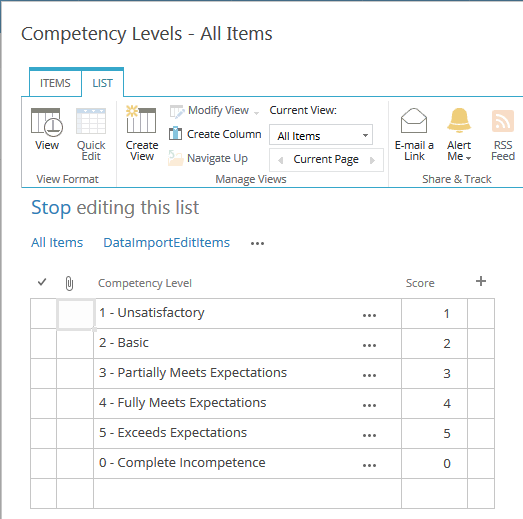During the competency assessment, each competency is assigned some score.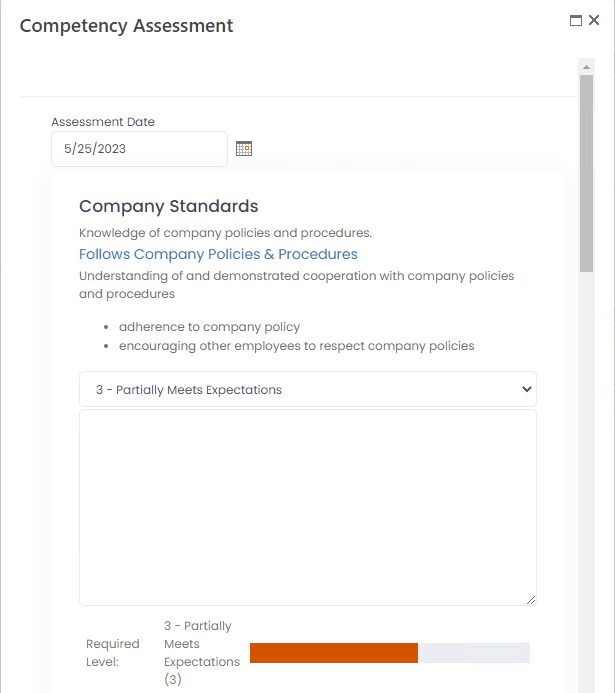The competencies can also have the required level that is defined when assigning competencies to the job roles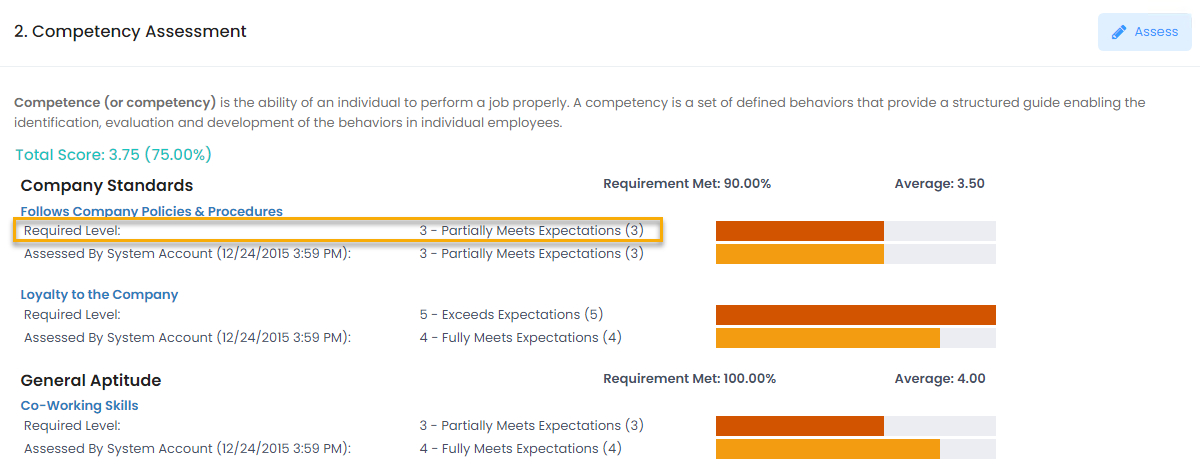The Total Score for the competencies can be calculated based on the competency assessment only or competency assessment in relation to the required level. This depends on the Total Score Calculation Method setting, where you can select one of the following options:

• Required Level Not Considered - the Total Score will be calculated based on the competence assessments only.
In this case, the maximum level score is considered to be 100% and in relation to it, the percent for the assigned score is calculated. For example, the maximum score is 5 (Exceeds expectations) and the competencies were assessed as 3 (Partially Meets Expectations) and 4 (Fully Meets Expectations). The total score will be 3.5, that is 70%.
• Required Level Considered - Capped - the Total Score will be calculated based on the competence assessments in relation to the competency required level, and the maximum score is 100%. For example, if the required level is 3, and the competency assessment is 4, the achieved score will be 100%.
• Required Level Considered - Not Capped - the Total Score will be calculated based on the competence assessments in relation to the competency required level, and the maximum score can exceed 100%. For example, if the required level is 3, and the competency assessment is 4, the achieved score will be 133.33%.
Note

The Total Score Calculation Method setting influences only competency assessments done during the performance reviews. During the other assessments, the competency required level won't be considered.

## Requirement Met

The competency requirements are defined for each job role. Based on employee's job role, the competency requirements are displayed in the employee competencies.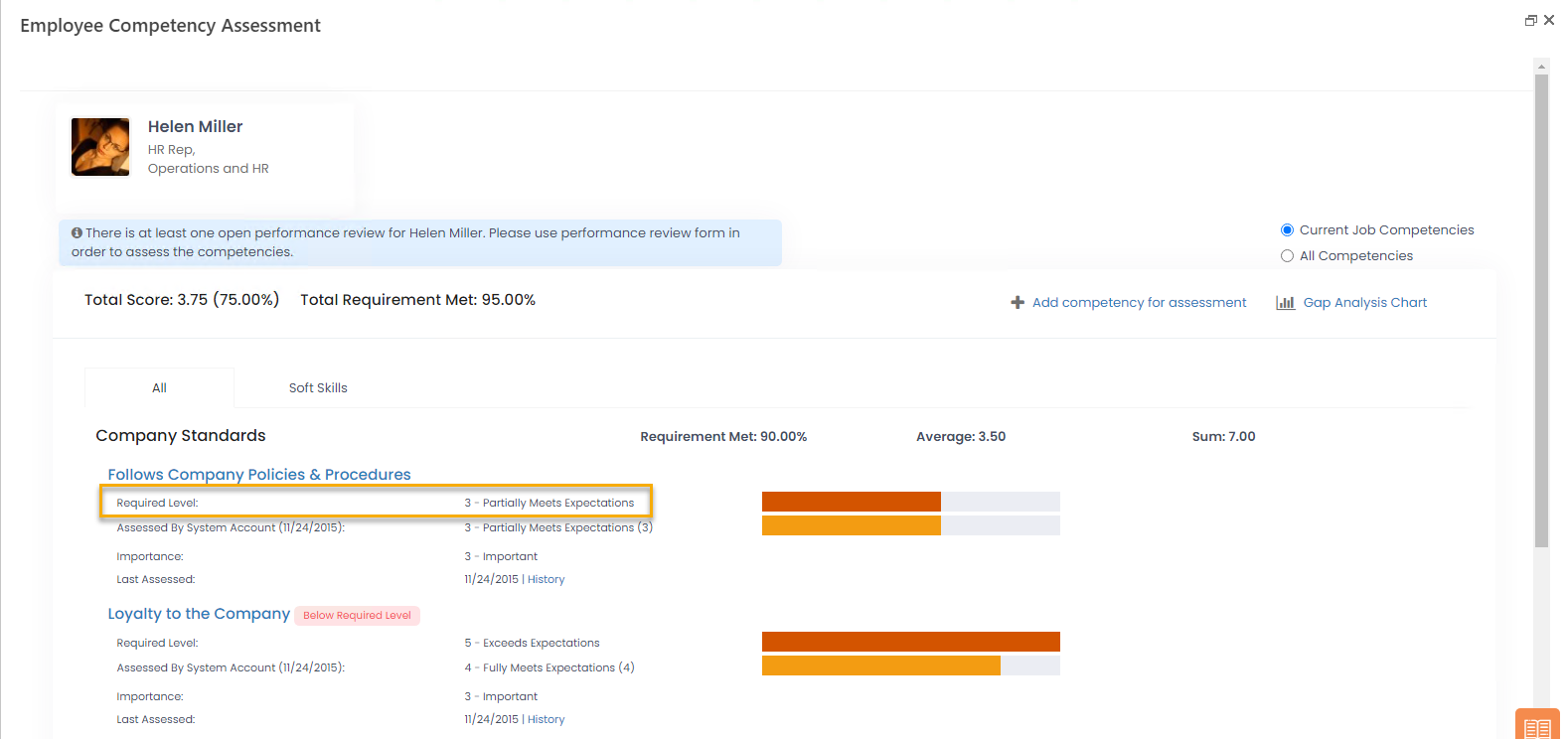The requirement met percent is calculated in relation to the required level, which is considered as 100%. The percent is calculated for each competency in the competency group and then the total Requirement Met percent is defined for the group. It is calculated as a sum of all the individual competency percents divided by the number of the competencies in a group.

For example, there are 2 competencies in a group. One competency has a required level of 5 and was assessed as 3, and another one has a required level of 4 and was assessed as 4. The calculation for the Requirement Met will be as follows: (60% + 100%) / 2 = 80%.

Note

Please note that the Total Score Calculation Method setting doesn't influence the Requirement Met score. Then Requirement Met score cannot exceed 100%. If the achieved score is more than 100%, it is still considered as 100% by the system.